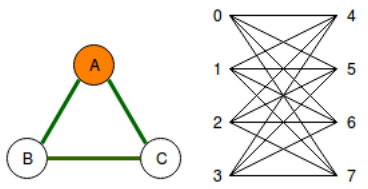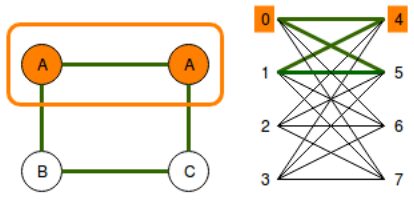# Difference between D-Wave Sampler and an Embedding Composite D-Wave Sampler?

What is the difference between `sampler1` and `sampler2`?

`from dwave.system.samplers import DWaveSamplerfrom dwave.system.composites import EmbeddingCompositesampler1 = DWaveSampler()sampler2 = EmbeddingComposite(DWaveSampler())`
` `

1 comment
• In the quantum processor (QPU), there is an array of qubits. Each of these qubits are labeled and are connected in a very specific way.

When using just the plain D-Wave sampler (without calling Embedding Composite), the user has to try and fit their graph directly into the quantum processor, in terms that the quantum processor can understand. (i.e. using the same qubit-indexing as the hardware, using only the connections available in the hardware)

Dealing with the quantum processor directly can be difficult as there limitations to the number of connections and to which connections a particular qubit can make. So instead of dealing with QPU directly, Embedding Composite takes the user's graph and transforms the graph so that fits it onto the QPU. Once the QPU has found a solution, Embedding Composite reverses the graph transformation and returns the solution in terms of the labelling used in the original graph. Short answer: Embedding Composite is like a translator between the original graph connections and the actual hardware connections.

For example, suppose we want to embed the triangular graph (left figure), directly onto a set of qubits on the QPU (right figure).It would be impossible to do so because there is no set of three edges on the right figure that would form a triangle. So instead, a "cloning" process is necessary in order to embed the triangular graph onto the QPU. Thus, in the example below, qubits 0 and 4 are strongly coupled together and act as one.